## Tables index

#### Peter D. Hart

American Journal of Medical Sciences and Medicine. 2019, 7(5), 190-197 doi:10.12691/ajmsm-7-5-2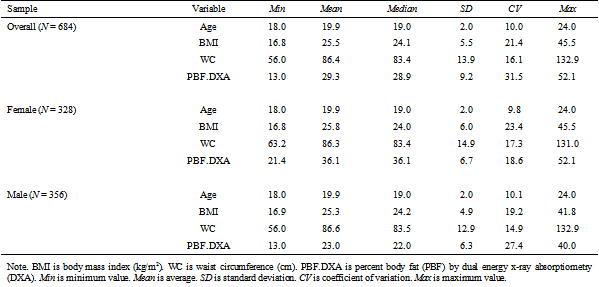• Table 1. Descriptive statistics for entire sample on prediction equation variables by sex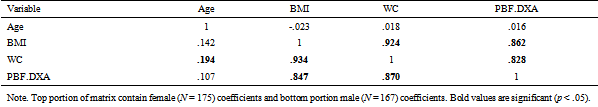• Table 2. Correlation matrix for training sample prediction equation variables by sex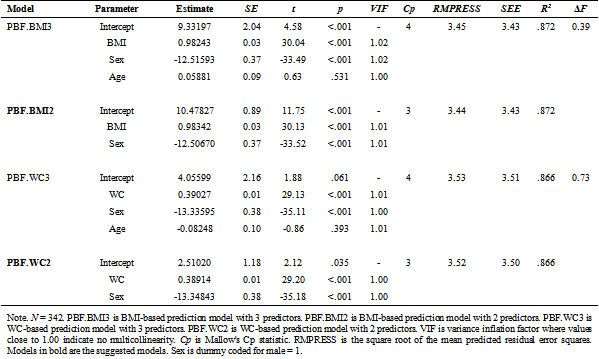• Table 3. Training sample regression models estimating criterion values of PBF (PBF.DXA) using BMI and WC as main predictor variables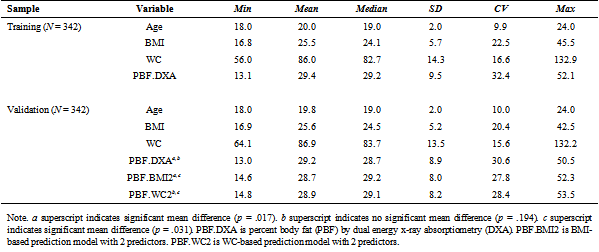• Table 4. Descriptive statistics on prediction equation variables by random split samples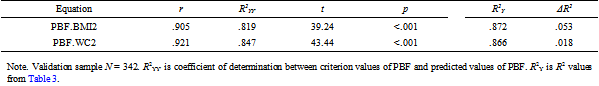• Table 5. Cross-validation coefficients between criterion values of PBF (PBF.DXA) and predicted values of PBF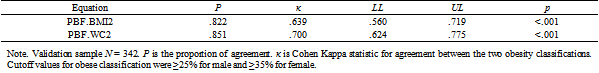• Table 6. Cross-validation coefficients between criterion classification of obesity and predicted classification of obesity